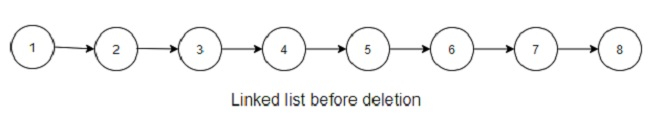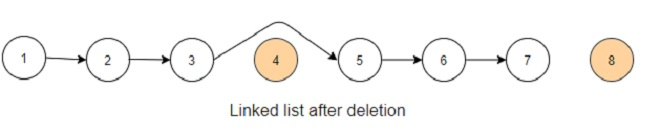# Program to delete n nodes after m nodes from a linked list in Python

Suppose we are given a linked list that has the start node as "head", and two integer numbers m and n. We have to traverse the list and delete some nodes such as the first m nodes are kept in the list and the next n nodes after the first m nodes are deleted. We perform this until we encounter the end of the linked list. We start from the head node, and the modified linked list is to be returned.

The linked list structure is given to us as −

Node
value : <integer>
next : <pointer to next node>

So, if the input is like elements = [1, 2, 3, 4, 5, 6, 7, 8], m = 3, n = 1, then the output will be [1, 2, 3, 5, 6, 7, ]Every node after 3 nodes is deleted in this process, so in the end the linked list will look like below −To solve this, we will follow these steps −

• q := 0

• p := 0

• while curr is not null, do

• q := q + 1

• if q is same as m, then

• for i in range 0 to n, do

• if curr.next is not null, then

• curr := next of curr

• next of prev := next of curr

• q := 0

• prev := next of prev

• curr := next of curr

## Example (Python)

Let us see the following implementation to get a better understanding −

Live Demo

class ListNode:
def __init__(self, val=0, next=None):
self.val = val
self.next = next

def make_list(elements):
for element in elements[1:]:
while ptr.next:
ptr = ptr.next
ptr.next = ListNode(element)

print('[', end = "")
while ptr:
print(ptr.val, end = ", ")
ptr = ptr.next
print(']')

q = 0
p = 0

while curr:
q += 1
if q == m:
for i in range(n):
if curr.next is not None:
curr = curr.next
prev.next = curr.next
q = 0

prev = prev.next
curr = curr.next

elements = [1, 2, 3, 4, 5, 6, 7, 8]
print_list(res)
[1, 2, 3, 4, 5, 6, 7, 8], 3, 1
[1, 2, 3, 5, 6, 7,]